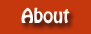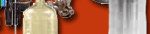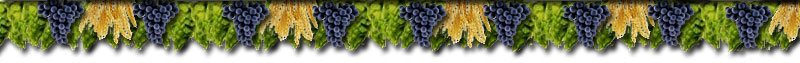Converting Extract to Grain

Converting Extract to Grain

Step 1

Arriving at your Efficiency Using a Known 5-Gallon Recipe

Given Information:

1 pound of dry malt extract in one gallon of water yields roughly a 1.045 S.G. (Specific Gravity).

This is considered optimum efficiency. So, 5 pounds of dry malt extract in 5 gallons of water would yield the same 1.045 S.G. (Specific Gravity).

For simplicity sake, the S.G. (Specific Gravity) in the formula below is expressed in only the thousandths... 1.045 becomes 45 (also called Brewers Units, abbreviated Bu).

Formula Variable Values:

S= The OG (Original Gravity or Starting Gravity) of a known 5-gallon recipe that you have brewed using your present all grain Equipment and Technique.

G= Pounds of grain used in a known 5 gallon recipe that you have brewed using your present all grain Equipment and Technique.

(Note: S and G should be from the same recipe that you have brewed using your present all grain Equipment and Technique.)

Using a Given OG and Pounds of Grain to Arrive at an Efficiency

Formula: 5S:G45

If you used 9.5 pounds of grain and arrived at OG of 56 the result will be 280:428

or 65% Efficiency.

 Or put it another way: Maximum/optimum OG for 9.5 lbs grain is 45Bu x 9.5 = 428Bu in 1gal or 428/5 in 5 gals = 85.6Bu so a result of 56 in 5 gals represents an efficiency of 56/85.6 = 0.65 or 65% efficiency

Step 2

Using your Efficiency to Determine the Value of One Pound of Grain Relative to One Pound of Extract

Given Information:

1 pound of Liquid Malt Extract (LME) in one gallon of water yields roughly a 1.035 S.G. (Specific Gravity).

1 pound of Dry Malt Extract (DME) in one gallon of water yields roughly a 1.045 S.G. (Specific Gravity).

Note: For simplicity sake, the S.G. (Specific Gravity)  in the formula below is expressed in only the thousandths... 1.045 becomes 45. (also called Brewers Units, abbreviated Bu)

Formula Variable Values:

B= Value in Specific Gravity of Grain relative to Extract (pound of per gallon of water)

X= Specific Gravity of Extract  (one pound of extract per gallon of water)

E= Efficiency expressed in a decimal (% of  grain that is converted to sugars. This varies due to your equipment and technique.)

Formula:

B=XE

Using the Formula and LME to Arrive at B:

B= 35*.65

Solved B = 22.75

Using the Formula and DME to Arrive at B:

B= 45*.65

Solved B = 29.25

 In other words, for a 65% efficient process instead of extraction 35Bu per 1 lb of LME in 1 gal of water we obtain 22.75Bu.   Similarly, for a 65% efficient process instead of extraction 45Bu per pound of DME in 1 gallon we obtain 29.25Bu.

Step 3

Arriving at Pounds of Grain to Substitute for 1 Pound of Extract

Note: For simplicity sake, the S.G. (Specific Gravity) in the formula below is expressed in only the thousandths... 1.045 becomes 45. (also called Brewers Units, abbreviated Bu)

Formula Variable Values:

P= Pounds of grain. (pounds of grain to replace each pound of extract per gallon)

B= Value in Specific Gravity of Grain relative to Extract (pound per gallon of water)

X= Extract Specific Gravity (one pound of extract per gallon)

Formula:

BP=X

Using the Formula and LME to Arrive at P:

22.75P=35

Solving for P results in the pounds of grain per gallon to replace 1lb of LME per gallon.

P=1.521

 In other words, to obtain 22.75Bu with grain instead of LME we need 35/22.75 = 1.521 lbs of grain.

Using the Formula and DME to Arrive at P:

29.25P= 45

Solving for P results in the number of pounds of grain per gallon to replace 1lb of DME per gallon.

P= 1.556

 In other words, to obtain 29.25Bu with grain instead of  DME we need 45/29.25 = 1.556 lbs of grain.

Recipe Examples:

Example 1: LME Recipe Example Using the Result Above (65% efficiency):

Now if you have 6.6 pounds of liquid malt extract in a 5-gallon recipe... you will have 6.6/5 = 1.32 pounds of LME per gallon.  So you will have to increase the grain per gallon by 32%.

Multiply 1.521 by 1.32 giving you 2.01 pounds per gallon.

You will need to have 2.01 pounds of grain per each pound of extract in the recipe. Or 13 pounds 4 ounces. (6.6 x 2.03) to replace the 6.6 pounds of extract in this recipe.

Example 2: DME Recipe Example Using the Result Above (65% efficiency):

Now if you have 6.0 pounds of liquid dry malt extract in a 5-gallon recipe... you will have 6.0/5= 1.20 pounds of DME per gallon.  So you will have to increase the grain per gallon by 20%.

Multiply 1.556 by 1.20 giving you 1.87 pounds per gallon.

You will need to have 1.87 pounds of grain per each pound of DME in the recipe. Or 11 pounds 4 ounces. (6.0 x 1.87) to replace the 6.0 pounds of extract in this recipe.